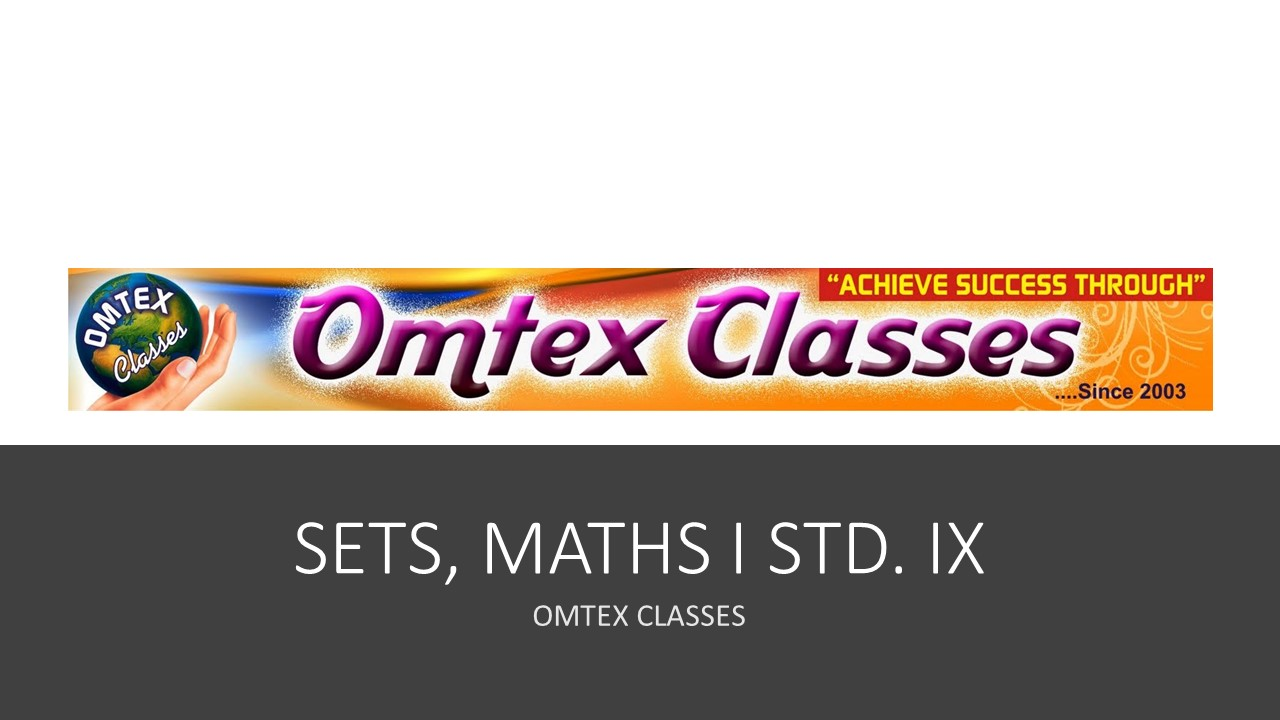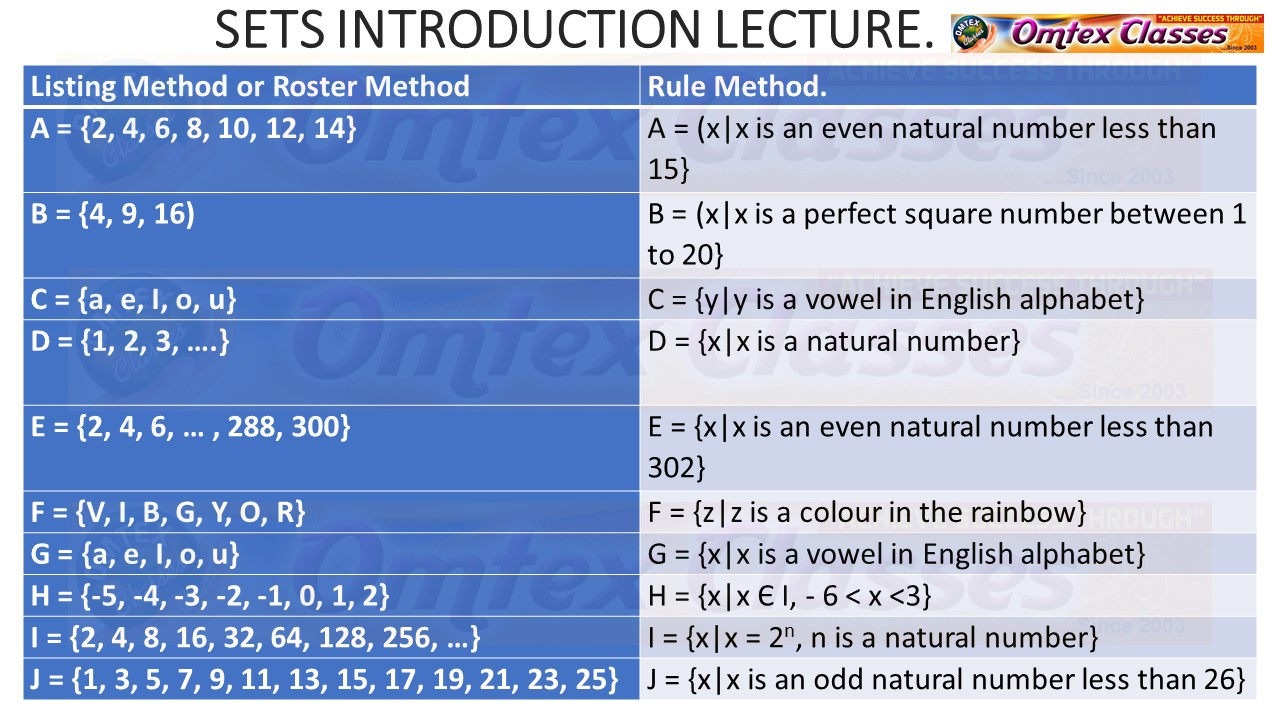### SETS IX MATHS I, INTRODUCTION, MAHARASHTRA STANDARD 9. Video Explanation + NotesLink for Better Studies in Detail: https://www.omtexclasses.com/2019/03/sets-ix-maths-i-introduction.html
In day-to-day life, we generally talk about a group or a collection of objects. e.g. a pack of cards, a group of students, a group of friends, etc. 1. The well-defined collection of objects is called a set, 2. Capital English letters A, B, C, etc. are used to denote a set. 3. The individual object of the set is known as the member or an element of the set. 4. The elements of the set are denoted by lower case letters a, b, c, etc.5, If a is an element of set A, then it is written as a ∈ A, and is read as 'a is an element of set A' or 'a is a member of set A' or 'a belongs to set A'. 6. If a is not an element of set A, then it is written as a ∉ A. The symbol ∉ stands for 'is not an element of' or 'is not a member of'. Thus, it is read as 'a is not an element of set A' or 'a is not a member of set A' or 'a does not belong to set A'. Let us see the set of numbers. (a) A set of natural numbers. N = {1, 2, 3, ...} (b) A set of whole numbers. W = {0, 1, 2, .....} (c) A set of integers. I = {... -3, -2, -1, 0, 1, 2, 3, ....} (d) Q is the set of rational numbers. (e) R is a set of real numbers.